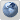Kimball Forum
Would you like to react to this message? Create an account in a few clicks or log in to continue.

# Rounding to 100%

3 posters##Rounding to 100%

We have a metric that calculates percentage of a day (ie asset was in status X for .25 days). For any given day the value should not exceed 1. There are cases where we are seeing rounding issues and the total `days` may equal 1.01 or .99 when it persists the record(s) to the fact. Looking for best ways to handle these scenarios to make sure the value always equates to one if we are representing a full day. Seems like we would need to create a process at the end of the load that would identify any cases where the some of `days` for any given day was greater then 1 and if 1.01 would choose one of the records and subtract .01 from that record.....I am not sure how efficient this type of approach would be thought and wanted to see how other folks are handling this situation. We have a great deal of metrics that can exhibit this behavior.

Thanks.....

drudini

Posts : 1
Join date : 2011-05-03

##Re: Rounding to 100%

Frankly, I would fix your code and be done with it. The most likely problem is you are using binary floating point values. Such data types are unable to properly represent decimals. Use a scaled numeric data type.ngalemmo

Posts : 3000
Join date : 2009-05-15
Location : Los Angeles##Re: Rounding to 100%

Even with an exact decimal data type you can still run into problems if you allow rounding errors to accumulate. For example, if a widget is three for a dollar, how much is each widget to the nearest penny? If we take (\$1.00 * 1/3) we get \$0.33 + \$0.33 + \$0.33 = \$0.99 Ooops, the total doesn't balance out!

The way I have handled this in the past is to calculate the fraction allocated so far rather than the fraction for each item. With the above example, instead of calculating (1/3), (1/3), (1/3) which allows rounding errors to accumulate, I would calculate (1/3), (2/3), (3/3) and then for each item sutract out the amount previously allocated, i.e. (1/3 - 0), (2/3 - 1/3), (3/3 - 2/3). Rounded to the nearest penny it would be (\$0.33 - \$0.00),(\$0.67 - \$0.33),(\$1.00 - \$0.67) resulting in widget costs of \$0.33 + \$0.34 + \$0.33 = \$1.00 Matches to the penny!

If you think of a pie chart, instead of calculating each slice of the pie individualy, calculate how far around the pie you have come, then subtract out the amount previously allocated. This prevents rounds errors from accumulating as you work your way around the pie, and you will always end up whole after the last slice!

This approach will work anytime you are dividing a fixed whole into pieces and need the sum of the pieces to excactly equal the whole. The pieces do not need to be the same size, but you do need to keep track of how much was previously "allocated". The rounding "bobble" will never be more than 1; in the above example there is a 1 penny difference between the prices of the widgets.

Last edited by VHF on Wed May 04, 2011 12:16 pm; edited 1 time in total (Reason for editing : added comma)

VHF

Posts : 236
Join date : 2009-04-28
Location : Wisconsin, US

##Re: Rounding to 100%

When you are doing calculations like that, you need to do them at a higher precision to avoid the issue. If the database columns do not have the necessary precision defined, most DBMS's have a cast function that allows you to convert values to a higher precision. For example casting from a NUMERIC(15,2) to NUMERIC(19,6). Significant rounding then doesn't occur until the final value is presented.ngalemmo

Posts : 3000
Join date : 2009-05-15
Location : Los Angeles##Re: Rounding to 100%